## CHEMICAL REACTIONS

#### Introduction

The first six modules of these chemistry courses deal with substances and their properties, where the next modules ask your attention for changes of properties when substances change.
In a chemical reaction you have reactants changing into products; new substances are made with new properties.
Often we refer to physical changes, like evaporation, as temporary changes, and chemical changes, like in reactions, as permanent changes.
Attention: it is not always easy to make this distinction.
The topics of this module: behaviour of particles in a chemical reaction, the reaction equation, reaction calculations, energy and energy diagrammes, reaction rate and the different types of reactions.

Question 1
Cooking patatoes, various processes take place. Explain what kind of processes.

Question 2
Dissolving a salt in water is a physical or a chemical change?

Question 3
Give some chemical reactions that might have taken place during billions of years, since start of the world.

#### Content

 1. General concepts 1.1 Regrouping of particles 1.2 Reactants and Products 1.3 The reaction Equation 1.4 Reaction calculations 2. Chemical reactions and Energy 2.1 Introduction 2.2 reaction energy; endotherm & exotherm 2.3 Activation energy 2.4 reaction steps and their energy changes 3. Reaction rate 3.1 Introduction 3.2 reaction mechanism / effective collisions 3.3 De reaction order 3.4 Influencing reactions 3.5 Diagrammes 4. Reaction types 5. Chemical Thermodynamics 5.1 Spontaneous and non-spontaneous reactions

## 1. Chemical reactions in general

### 1.1 Regrouping of particles

At a chemical reaction no particles are lost or appear spontaneously from nothing; during a chemical reaction, particles changes their character and/or there position.
 The participating particles do regroup themselves.

You can approach this in another way: during chemical reactions, the particles are removing from oneanother, and after that, they approach each other again to connect in a new way.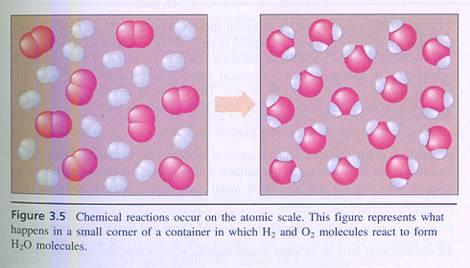Question 4
Statement: "At a chemical reaxction the total number of particles does not change."
Is this statement true? Explain.

Question 5
Describe what happens with the participating particles in the following reactions:
1. photosynthesis (water + carbondioxyde produce glucose and Oxygen (use models or good drawings)
2. the precipitation of Silver chloride (starting with NaCl(aq) and AgNO3(aq))

In general we may say: reaction between particles only take place at the moment that those particles meet, have contact. The particles must collide.
And then more: not every collision leads to reaction; that collision must take place in the very right way, must be effective and have sufficient energy.
The particles can be atoms, ions or molecules, and can be small or big.

Question 6
A macromolecule can react with a small ion in the mol-proportion 1:1
Make a nice estimation of the mass proportion of the reactants, in such a case.

### 1.2 Reactant, reagent, product, side product, overdose.

Substances in a chemical reaction react or are made, always in a fixed proportion. A reaction between reactants continues as long as there are substances available. It stops when one of the reactants is gone.
The rest just remains, and can no longer react; it was present in 'overdose'. You must understand that it is wise to calculate, on beforehand, how much you need of every substance in a reaction.
Only with good calculations you can avoid that any substance remains without options. On top of that, the remaining substance could easily complicate the reaction. Calculating well is a first condition in a chemical reaction.
Another disadvantage of badly / no calculating (reaction calculations) is that the products at the end can be polluted.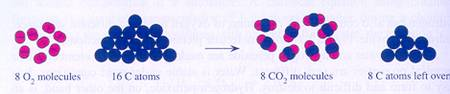Question 7
Many reaction occur in the presence of substances that do not really participate in the proper reaction. An example: precipitation reactions.
1. Explain that precipitation reactions occur in the presence of non participating substances.
2. Name other examples of reactions occurring in the presence of non participating substances.

#### Side products

In the lab or in the chemical industry, we like to produce chemical products. A general problem is, that, besides the aimed main products, many times always are formed so called side products, mostly unavoidable because the reaction does produce also those substances.
Maybe this will not be a problem, but often purification is needed, and that can be rather complicated. Its a nasty problem.

Question 8
Precipitation reaction:  NaCl(aq) + AgNO3(aq)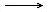AgCl(s) + NaNO3(aq)
in this reaction, the reactants Ag+ + Cl- change into the product AgCl(s).
Apart from the meant final product, side products are made: NaNO3(aq). This is a simple example of a side product.
How could you, in this case, get the pure end product?

### 1.3 The reaction equation

Changes during a chemical reaction are indicated with an arrow:, the reacting substances before, de producst after.

ReactantsProducts         (reaction equation)
Sometimes you can write a reaction in words (like: Calcium with waterChalk and Hydrgen),
but normally we use the chemical symbols for each participating particle and we show the right mol proportion as also the phase of each component.

The total number of participating elementary particles (atoms and ions, but also protons, neutrons and electrons) does not change during the reaction.
You start a reaction of course with the right formulas; but if they are there, you must take care that the number of particles per element is equal at both sides of the arrow. No atoms disappear, nor are created during a normal chemical reaction.
With 'coefficients' we 'balance' the equation: for example, 2 molecules of this react with 3 ions of that, etcetera.

These coefficients show us: the mol proportion.

Besides that, you always must have a look at the electric charge at both sides. That also may not suffer any change. If you notice any charge change in the equation, something is wrong.

In general:
1. You take care of the right formulas of the participating substances;
2. You balance the equation with coefficients;
3. You add the phase of every substance: (s) (l) (g) (aq); [(aq) is in fact not a real phase!]
4. Of ions you write normally the ionic formulas in the equation (but not always!)
Only if calculations are to make (reaction calculations), than it is advisable to write the empiric formulas (not de ionic), for the substances built up of ions.
Question 9
Give the balanced equation of the following reactions, and give also the mol proportion of the reactants.
1. The photosynthesis
2. Calcium reacts with water and forms 'extinguist chalk' and a gas.
Give here two reactions: one in ions and one in empiric formulas.
3. The precipitation at mixing Sodiumhydroxyde(aq) and Copper(II)sulfate(aq). (also with ionic formulas and with emiric formulas)
4. the production of ammonia gas from the elements
5. The decomposition of water
6. Chalk with hydrochloric acid
7. the production of Iron(II)sulfate from the elements
8. the combustion of natural gas
9. the combustion of petrol
10. the donation of two electrons by an atom of Calcium
If possible, imitate the reaction with models.

Very special is the nuclear reaction. There the elements change, what never happens in normal chemical reactions.
Yet the normal rules are to be applied in nuclear reactions: number of particles and charge at both sides of the arrow.

Examples: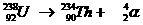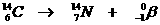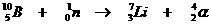Question 10
The rules for ordinary reaction equations are the same for nuclear reactions.
Check that.

### 1.4 reaction calculations

Whereever you apply chemistry, for example in a lab or in a factory, making a product needs knowledge of the needed
How much product you want? How much reactant is needed therefore? In what proportion you must mix the components?

So, well calculating before starting is not only important to get the right product, but shows also your economic understanding. It is stupid to use too much of any reactant.

Question 11
Somebody wants to start a soap factory. Needed for the process are: vegetable oil, salt, sodium hydroxyde, water, energy, buildings, etcetera.
Describe in short the plan of operations.

The application of reaction calculations can only be done by someone who knows to deal with the concept of MOL (see also module 5).
It is very important to be capable to make calculation conversion from MOL to grammes and v.v. Besides that, you must know what density is of solids and liquids.

Remeber the following equation: d = g/v?

Gases also have a density, but with a very different definition than density of (s) and (l). In a next paragraph we will meet that.

Already good to know about gases: whatever gas you take, with big or small molecules, one mol of it always has the same volume (measured at the same temperature and pressure). Or the other way round? if you take 1 lieter of a gas, measured at a fixed temperature and pressure, this 1 liter has the same number of moles, no matter what gas you take.
MOL and volume of gases are directy connected. What is impossible with solids or liquids, can be done with gases: the coefficients of gases in a reaction equation can be read as MOL and as liters.
The mol proportion = de volume proportion.

Question 12
What volume has 1 mol gas at a pressure of 1 atm. and a temperature of 0oC ?

If methane reacts with Oxygen in the proportion 1:2 (one mol methane reacts with 2 mol Oxygen), this means that simultaneously and automatically, under the same conditions, 1 liter of methane gas reacts with 2 liter Oxygen gas.

You must know the concepts of "molarity" and concentration. You will meet these very often in reaction calculations.

For example: 10 ml 0,1M HCl reacts with one or another substance. You must be able to apply these data in the calculation. You must know very well this way of writing.

A reaction calculation always starts with a well balanced reaction equation, that clearly indicates the mol proportion.

Question 13
Calcium reacts with water, whereby calcium hydroxyde and a gas is formed.
In what mol proportion react the reactants, and calculate their mass proportion.

The calculation in question 13 is an example to show the advantage of using empiric formulas instead of ionic formulas.
Calculations deal with real and practical things and in practice you don't get ions from a bottle, but complete neutral substances.
So, even if only Chloride ions participate in the reaction, you will measure salt (NaCl) on your balance.

Again: it is wise, for good understanding of the practice, to indicate if the substances are (s), (l) or (g) (or (aq)).
Ca(s) + 2H2O (l)Ca(OH)2(aq) + H2(g)
The reactants react in the mol proportion 1 : 2

Att.? That mol proportion shows in what proportion the substances react; that can be very different than the composition wherein the substances were mixed in reality. If you do not mix as well as possible in the right mol proportion, unused substance will remain (and complicate).

Question 14
A mix of 100 mol of Hydrogen and 1 mol of Nitrogen do not react in the proportion 100:1, but yes in the mol proportion defined by the equation.
How many mol of product can we expect in this case?

 Important rules for resolving a reaction calculation: 1 Use balanced reaction equation and add the phases. 2 Underline the data, the given data and those asked for. The other substances (without any data) are not needed in the calculation. Sometimes the data are directly given, sometimes indirectly. The calculation is continues with only the underlined substances. 3 write down the mol proportion 4 Where needed, convert the mol into the right units (to be found in the data and in the required answer) 5 Introduce a conversion factor to arrive at the real amounts, given in the data. That's how you finish the calculation.
Att.: It is absolutely necessary that you continues in every step with all (underlined) substances, to assure that you know what you are doing. Only then the calculation remains a real thing, and not an abstractum.

EXAMPLE:
Question 15
 Calculate mass and volume (standaerd conditions for gases) of carbon dioxyde produced in the complete combustion of 4,01 g methane? 1 CH4(g) + 2 O2(g)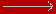CO2(g) + 2H2O(g) 2 Underline the substances with data. CH4(g) + 2 O2(g)CO2(g) + 2H2O(g) 3 So, 1 mol CH4(g) reacts with 1 mol CO2(g) (proportion 1:1) 4 16 gramme CH4(g) produce 44 gramme CO2(g) (here we apply the molecular masses) 5 in reality we do not have 16 grammes, but only 4 grammes for combustion.
To be introduced a factor of 4/16. (in this case we can simply divide by 4)
4/16 x 16 gramme CH4(g) produce 4/16 x 44 gramme CO2(g)

to finish: standard condition mean: at temp = 25oC and pressure of 1 atm. Then 1 mol gas = 22,4 liter

1/16 x 44 = 11 gram CO2(g) is produced,

that equals 4/16 mol = 4/16 x 22,4 liter CO2(g) = 5,6 liter Goto answer 07-15

Again the rules for resolving the reaction equation:
1. A balanced reaction equation + phases.
2. Underline the substances about which you have data or you must find answers.
3. Note the MOL proportion
4. If needed, convert MOL into other units.
5. Introduce a calculation factor, to reach the real amounts (see data).

Question 16
1 g Sodium is burned in 100 liter of air(0o C and 1 atm.).
Calculate, in grammes, how much Oxygen will remain.

Question 17
Ore of Iron contains 75% iron(III)oxyde.
Calculate the mass of the Iron that can be obtained from 1 ton of ore.

Question 18
Someone is using natural gas (methane) to make water boil during 15 minutes, in order to kill possible bacteria.
She used therefor 5 g of the gas.
Calculate the mass, in grammes, of the needed Oxygen for this whole process.

Question 19
To burn 1.3 mol natural gas, how many mol of Oxygen are needed? (all measured at standart conditions)

Question 20
Calculate the amount of ammonia and Oxygen (in grammes) needed to produce 3.00 g NO. A side product is water.

Question 21
Concentrated sulphuric acid contains 96% pure H2SO4. The rest (4%) is water.
Calculate the number of mols H2SO4 in one lieter concentrated sulphuric acid, knowing that the density of it is 1,84 g/cm3.

Question 22
3 mol of chlorine and 150 gramme of Iron react with each other and form iron(III)chloride.
Which is the limiting substance (is completely consumed?): Chlorine or Iron?

Question 23
Nitrogen and Hydrogen react in a direct reaction with each other to produce the prodect ammonia, in an industrial reactor with catalysts.
The result is 1000 grammes of product (NH3) with a yield of 97,8%
The molecular mass of ammonia = 17
How many mols reactant are needed?

## 2. Reactions and energy

### 2.1 Introduction

Some reactions occur totally spontaneous, do not need anything; example: natural radioactivity. But in most cases a chemical reaction needs some 'action' to start.
This action can be various: lighting, heating, moving, joining, electricity, more...

Question 24
Describe the processes that occur when lighting a match.

### 2.2 reaction energy; exothermic & endothermic

Every substance has a certain amount of (chemical) energy. The most suitable place for this chemical energy is in the bondings between the atoms of the substance. It is there that change can take place.
Bondings can be very rich in energy, for example the activated bondings in ATP or ADP (the energy carriers in living organisms).
But most bondings are normal, representing an amount of energy that we can find in tables.
If bondings have little energy, they probalby will be more stable.

In a chemical reaction, the reactants change (they had their own amount of energy in there bonds) into product (also having their own amount of energy, but different from that of the reactants).
So:

During a cemical reaction change in chemical energy will be realised.

There are three possibilities:
 I The products have more energy than the reactants In this case de products have gained energy; this is only possible when the system gained energy from outside (ΔH > 0). II The products have less energy than the reactants Here the reactants lost energy; the system has donated energy to the outside environment. (ΔH < 0). III The products have the same energy as the reactants In this case there is a chemical equilibrium. (ΔH = 0).

#### Energy diagrams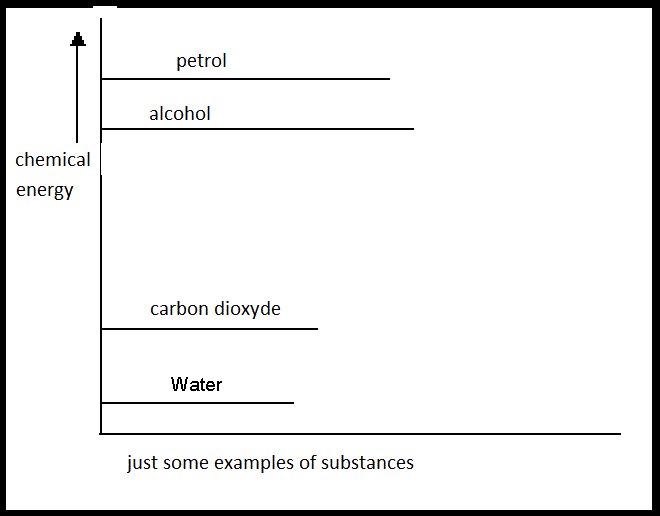If petrol reacts with Oxygen (these are two rather energy rich substances) than the products water and carbondioxyde are formed (two substances with very little internal energy).
So in this example the system lost a lot of energy during this combustion. The lost energy does not disappear (energy never get lost). This energy goes outside, in this case as heat.
The difference in energy of products and reactants is also called: the reaction energy with the symbol: ∆H.
If energy is delivered by any system, ∆H has a negative value. We talk about an 'exothermic' reaction.
If the system gains energy, than ∆H has a positive value. We talk about an 'endothermic' reaction.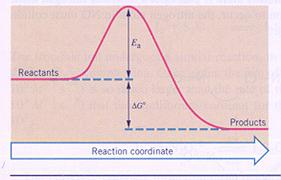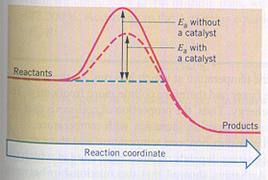Reactants change to products, with or wihtout catalyst.
ΔGo gives the reaction energy for what often is used the symbol ΔH.
Eais the activation energy.

Question 25
Condensation of water vapor, will that be an exothermic or an endothermic process? Explain your answer.

Question 26
Construct an energy diagram for 'the combustion of petrol'.

In a way you may say: there are "weak substances" (with little reactivity) and "strong substances" (with a lot of reactivity).

#### Explosives

Explosive substances not only have a lot of internal, chemical energy, but they also start easily and will produce lots of gaseous products.
The reactants (the explosives) are solid or liquid, so during the reaction their is an enormous increase of volume in very short time + lots of energy coming out.

TNT = trinitro toluene forms the products: NO2, CO2 and H2O. All gaseous products.
During its explosion, only one gramme of TNT can cause, in a splitsecond, several liters of gas.

### 2.4 Reaction steps and their energy

#### Energy of chemical bonds

If atoms are connected in a chemical way (a bond is made), there is always delivery of energy (exothermic). The system loses energy. The value of ΔH has a negative sign.
The same amount of energy is needed to break such a bond, just to remove those atoms from each other. Then ΔH will get a positive sign.
There are tables with bonding energies. You may read such a table in two ways:
1. If bondings are made, when atoms come togheter, then you give the amount a minus sign.
2. If bondings are broken, when atoms leave each other, then you give the same amount a plus sign.

Question 27
The bondings energy of the Nitrogen molecule is very high. Explain that.

Every step of a reaction comes with energy changes, because every reaction step will change something in the particles and/or the bondings.

General rule:

Breaking bonds, separate particles, costs energy, is endothermic.

Making bonds, join particles, creates energy, is exothermic.

or

Separate costs energy and join gives energy.

[So if you want to economise energy, stay toghether!]

The fundamental steps in a chemical reaction are: breaking of bonds and making new ones.
To understand what happens in a chemical reaction, always try to imagine what the particles are doing.

Example:
Mixing two gases, ammonia and hydrogencloride, a white smoke appears of the solid (salt) ammonium chloride.

Barious reaction steps are part of the total reaction:

NH3(g) + HCl(g)NH4Cl(s)

An advantage is that the reactants already are gaseous, so, there is no need to separate the gas particles (the molecules NH3 and HCl) Een voordeel hier is dat de reagentia al gasvormig zijn, dus, het is hier niet nodig die gasdeeltjes eerst uit elkaar te halen. (Meestal, bij vaste stoffen en vloeistoffen dus, is dat wel zo en dat alleen al kost alweer energie).
Of each HCl molecule, first a H—Cl bonding must be broken, an endothermic process, costing energy.
Immediately after that, the new bonds N—H are made (result: NH4+) and that will deliver energyt, in an exothermic process.

The table with bondings energies indicates (tabel VI):

to break 1 mol H—Cl bondings costs 432 kJ

to make 1 mol N—H bondings gives 391 kJ

Only counting with these data, your conclusion could be: the total reaction must be endothermic (it costs more than it delivers).

But: we are not ready yet:
Ions are formed (1 mol ions NH4+ and 1 mol ions Cl-).
Those ions attrackt and join each other in an ionic lattice. This joining delivers a good amount of energy:
Making 1 mol of this ionic lattice of NH4Cl(s) delivers 400 kJ.
The total process turns out to be exothermic with a reaction energy of: 391 + 400 - 432 kJ = 359 kJ per mol ammoniumchloride product.

Question 28
If the elements Sodium and Clorine react with each other, a chemical reaction occurs with salt as a product (NaCl).
Based apon a well balanced reaction equation, and with help of the energy table (table VI) you must calculate how much energy will be freed or needed at the production of one mol of salt out of the elements.
Other data are:
• The atoms of Sodium are in a metallic lattice.
• The production of Sodium ions includes electron loss.
• the diatomic molecules of Clorine must first separate, and
• only then each atom Cl picks an electron to mak an ion.
• At the end, all new ions settle in an ionic lattice.

Question 29
The production of water out of element is a very strong exothermic process (explosion).
The product, initially made in gaseous form (vapor), becomes liquid at cooling.
H2(g) + ½O2(g)H2O(s)      ∆H < 0
Give all steps of this process until the liquid water; every step with its energy. And calculate also the total reaction energy.

In general, but certainly in the carbon chemistry, we may divide reaction steps in:
1. the start, or initiation of the reaction
2. the main reaction, the production of the product
3. end reactions, or termination
Steps 2 and 3 can cause side products.

## 3. The reaction rate

### The reaction rate

Every chemical reaction occurs with a certain rate V, that you can define:
V = the amount of reactant that disappears per second, or: the amount of product that appears per second.

The reason why one reaction occurs fast and the other slowly, that has to do with a number of factors, conditions that influence the reaction rate.

### 3.2 reaction mechanism / effective collisions

Every reaction has a reaction mechanism: The sequence of the different reactions steps.

During the steps intermediary products are formed. In the case of various steps, the total reaction rate depends on the slowest step.

Question 30
Explain why the slowest step determines the total reaction rate.

There are chemical reactions where the particles (e.g. molecules) - simply by possessing sufficient energy - decompose in a decomposition reaction.
In this case the reaction rate does not depend on the presence of other particles.
Such reactions are unimolecular, first order reactions: the particles do not need any collision with other particles to start a reaction. They can split spontaneously into different parts.

Question 31
The decomposition of ozon is calles a 'unimolecular' reaction, a first order reaction.
Try to explain.

Question 32
Analyse tho following graphic about the decomposition of 14C in a first order reaction.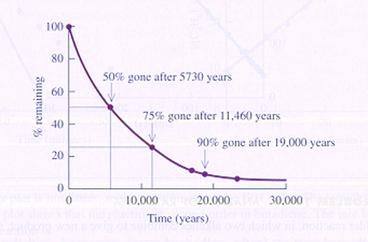Another example of a unimolecular reaction is the decomposition of N2O4.
With sufficient energy, the molecule will divide in two parts (NO2).
This very elementary reaction consists of only one step.

Question 33
Explain that the above mentioned decomposition reaction is the opposite of the reaction in the following figure: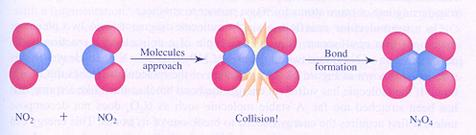effective collisions

Most chemical reactions are not unimolecular, but bimolecular: two particles must collide, and an effective collision leads to a reaction.
These are not elementary reactions; they have a complicated reaction mechanism with various steps.
A bimolecular reaction occurs not automatically in every collision. The collision should be 'effective'.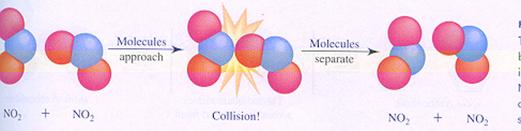a non effective collision

Below you see the images of two possible (different) mechanisms of the reaction between molecules of nitrogen dioxyde. You see the different steps.
In case I, two molecules collide; thats how the reaction starts.
In case II, one molecule splits and one of the products collides with another molecule.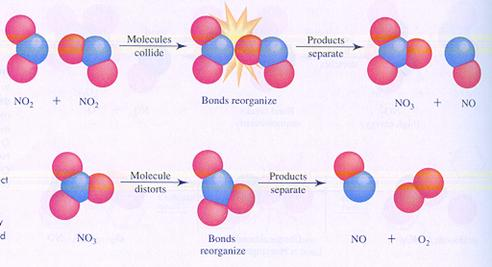case I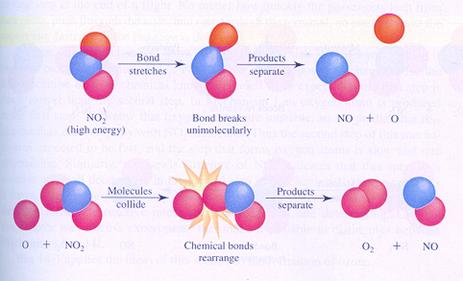case II

Question 34
Compare the two mechanisms and find the differences.

### The reaction rate

Every chemical reaction occurs with a certain rate V, that you can define:
V = the amount of reactant that disappears per second, or: the amount of product that appears per second.

The reason why one reaction occurs fast and the other slowly, that has to do with a number of factors, conditions that influence the reaction rate.

The reaction rate can be influences by several factors.
A formula has been derived for reaction rate wherein the most important and most influencing factors are included.
The rate depends on the concentration of the reactants [ ] , on the division/superficion of the substance (homo and heterogeneous), on the temperature (oC or K), and on the possible presence of a catalyst.

V ≈ [concentration] x division x energy of the particles x catalyst.

In this way, a formula is not very usefull. It is a formula in words. We want numbers and symbols.
You can simplify the formula keeping some circumstances constant during the reaction, in such a way that only the (not constant) concentration do remain in the formula.
Thus we get a mathematical formula:

V = k·[conc.]n

1. V: is the rate;
2. k: includes the whole of factors kept constant;
3. n: is the coefficient of the reactant as in the reaction equation;
4. In this way the concentrations of every homogeneous substance appears in the formula. Heterogeneous substances are left out;
5. A reactopm where k has a high value, has 'strong' reactants;ntia'
6. A reaction where k has a low value, has 'weak' reactants.

Question 35
Check the similarity between the data in the table below, and the graphic.
Looking well at the three graphics, what is your comment?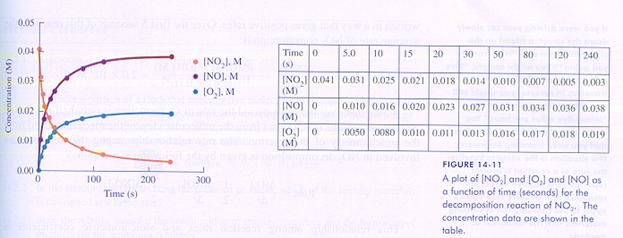Question 36
Why is it impossible to keep the concentration of the reactants constant during a reaction?

Question 37
Give the formula of the reaction rate of:
H2(g)+ Br2(g)2HBr(g)

Important with reaction rates is the reactivity of particles. There are particles with a very high reactivity, often called: 'radicals'. Those radicals are mad - generally spoken - under influence of light and have the peculiar thing that they possess unpaired electron(s).

Examples: Cl·       Br·      —  C — C — O·

Question 38
Explain why Ozon (O3) easily forms radicals.

Other attacking particles are those with charges. Some charged particles like (+, -, δ+ or δ-) show a great reactivity.
We distinguish two possibilities:
1. a negative particle attacks a positive particle = nucleophylic mechanism
2. a positive particle attacks a negative particle = electrophylic mechanism
An example to explore is the organic reaction between alkonoles and alkanoic acids in the presence of the catalyst sulphuric acid.
propanoic acid + ethanolethylpropanoate + water (sulfuric acid = the catalyst)

### 3.3 The reaction-order

The reaction order is defined as the final exponent of the concentrations in the rate formula V.
Normally, this exponent is derived from the coefficients in the reaction equation.

Question 39
What will be the order of the reactions below?
 Cl22 Cl· V = k*[Cl2] 2NO2N2O4 V = k*[NO2]2 CH3I + OH-CH3OH + I- V = k*[CH3I]*[[OH-]
Back to the reaction: H2(g)+ Br2(g)2HBr(g)
This seems to be a reaction of the second order (bimolecular), but rate measuring shows that the order of this reaction = 1½.
How to explain that?

The steps (including a radical mechanisme):
1. Br2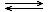2Br·       this equilibrium is reached very quickly
2. Br· + H2HBr + H·       this is the slowest step
3. H· + Br2HBr + Br·       this is the fastest step

Question 40
Give the first step, the last step and the main step.

Step 2, the slowest one, is determining the total rate:
 Vtotal =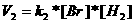Equilibrium 1 has as a condition: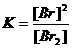If we combine the two (mathematical) equations, we can calculate: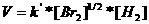which means that the reaction order must equal 1½ (see exponent)

### 3.4 How to influence chemical reactions

If reaction rate depends on the number of effective collisions, the next question is:
1. How to influence the number of collisions?
2. How to influence the effectivity of the collisions?

#### Factors that influence the number of collisions:

1. The number of present particles depends on the concentration, or (with gases) on the pressure. The more particles, the more chance to a collision.
Att: during a reaction the number of reacting particles automatically will decrease, so, of course the number of collisions will decrease, or: the reaction rate will decrease with time
2. The character and the situation of the reacting particles.
How are they present in the reaction? Are the divided in extremely small particles, or still stony? Have they little or great energy? Are they well mixed? etc.
3. The temperature of the reactants. This has immediately influence on the movement: higher temperature, more movement, more chance to collision.

Att.: We only talk about concentration when the division of the particles is homogeneous. In the case of heterogeneous substances, we do not speak of concentration.
In the rate formulas, heterogeneous substances are simply filled out with 1.

#### Factors that influence the effectivity of collisions

1. The presence of a catalyst. That one makes that the collision between particles is less by chance, less by accident; a catalyst accompanies, directs collisions.
The particles collide exactly on the right spot, for example, thanks to a catalyst that has a big influence this way (see also biocatalysts)
2. Again the temperature. Not only the movement of the particles will increase, but also their energy. And that can provide with higher effectivity.

att.: Every chemical reaction needs a minimum energy to start. Thus you can 'freeze' reactions:
As quick as possible lower the temperature under that minimum; then the rate comes at once to zero mol.sec.

Question 41
In the synthesis of ammonia according to Haber Bosch, the catalyst will help the step that divides N2-molecules into two atoms of N.
After that, a N-atom reacts with H2, etc.
1. Give at least two steps of this reaction and give also the order of every step.
2. Which one will be the slowest step and why?

#### Extinguish

Question 42
Which methode did you ever apply to extinguish a fire?

There are a number of ways to extinguish a fire:
1. cooling down until the temperature is under its minimum reaction temperature.
2. removing reactants, or prohibit the arrival of reactants
3. Add a helpinbg substance that itself cannot burn

### 3.5 Diagrammes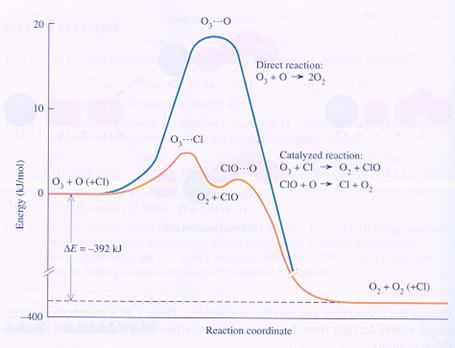#### (Bio)catalysts

In the diagramme you can see the chemical energy on the vertical axes and the course of the reaction on the horizontal axes:
Ozon + atoms of Oxygen normally form Oxygen, with help of the catalyst Clorine atoms.

Without clorine their is the need of an intermediate product with much energy (O3---O); the formatiuon of that one costs a lot of energy.
The atom of clorine can make a different intermediate product that costs less energy.

#### Temperature

In general you may say that the increase of temperature will increase the reaction rate.

Every 10 degrees increase will double the reaction rate.
(kind of general rule)
Inrease of temperature means automatically: more movement of the present particles; and this means more collisions between the reacting particles and more energy for each.

Question 43
Make two graphics in one diagramme:
1. The reaction rate at a certain temperature (for ex. 25oC) versus the reaction time in minutes.
2. The reaction rate of the same reaction at a temperature about 2 times higher (50oC).

#### Concentration; adding or removing substances

The following diagramme shows the concentrations of the reactants and products of a process that is about to reach equilibrium: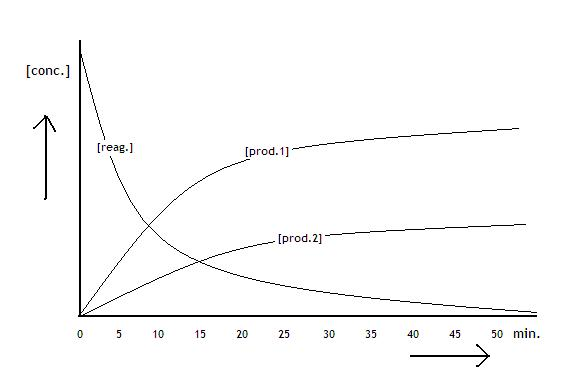Question 44
At moment 20 (minutes) we added 1 mol of reactant. How will continue the graphic?

Question 45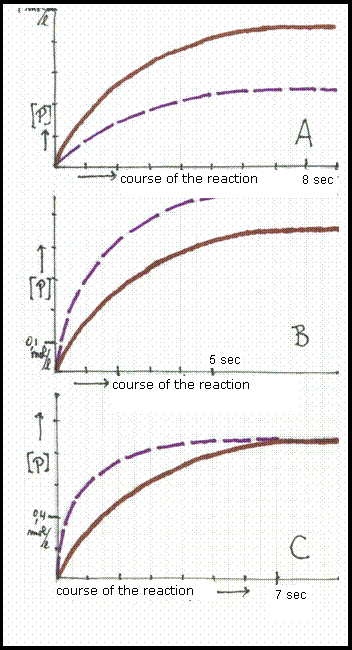The three diagrammesshow graphics: concentration of the product in mol/l versus course of the reaction in seconds.
Two graphics differ only in temperature, in such a way that the broken line shows the situation at the lowest temperature.

The reaction rate depends always on the concentration of the homogeneous substances. It will have immediate concequenses when changing one of the reactants. At adding one of the reactants, the rate must increase immediately (for some time!).

## 4. Reaction types

### 4.1 Introduction

Where all chemical reactions have lots of characteristics in common, yet we can distinguish various types.
Bonds can be broken or formed, completely or partially; electrons or other particles can be transferred from one substance to another; products can be made of a certain kind; reactions can be in equilibrium or not; or can be endothermic/exothermic. Etcetera.
This might be not the most exiting part of chemistry, but you must be capable to classify the chemical reactions.

#### Composition reactions

In these reactions, a substance is made from its elements. Most composition reactions are exothermic.
Many, if not all composition reactions can be divided in steps.
An example:
The production of water from the elements Hydrogen and Oxygen needs the following first steps:
• H22H
• O22O
• Only if loose Hydrogen and oxygen atoms exist, a water molecule can be composed.
• 2H + OH2O
• In general (but not always!) exothermic processes will dominate at the composition of substances from the elements.

With some help of the table with bonding energies, you can calculate the energy effect of the composition of water:
1. H2 + ½O2H2O
2. 1 mol H-H bonds are broken (cost how much energy?) sign +
3. 1 mol O=O bonds are broken (cost how much energy?) sign +
4. 2 mol O-H bonds are made (energy is delivered, how much? sign -

Question 46
Calculate in the same way the energy effect of the composition of one mol HCl from the elements.

#### Decomposition reactions

In these reactions a substance is decomposed into the elements. In general these reactions are endothermic.

#### Ionic reactions / precipitation reactions

Here are reactions in aqueous environment where (free) ions join to form insoluble ionic lattices.

Question 47
1. Every chemical reaction can be divided into composition steps and decomposition steps. Explain that.
2. Do you know other ways to divide the chemical reactions into two kinds? Which one?

#### Exothermic and endothermic reactions

A summery:
Exothermic is the reaction where the products have less chemical (internal) energy than the reactants.
endothermic is the reaction where the reactants have less energy than the products.

#### Acid base reactions

Reactions where H+-transfer takes place, from an acid (donor) to a base (receptor)

#### Redoxreactions

Reaction with electron transfer: one or more electrons go from the reductor (donor) to the oxydator (receptor)
If that oxydator happens to be oxygen, we may talk about combustion (direct or indirect).

#### Hydrolysis and Condensation

In these reactions water participates. Water can be used in hydrolysis and is be liberated in condensation.
Splittin a molecule in two parts, where water is used = hydrolysis
Joining two molecules, where one new molecule is produced and where water is liberated as a product = condensation
Hydrolysis and Condensation are each others opposite.

There is also polyhydrolysis and polycondensation, for example in the production or the decomposition of proteins an of starch.
polyethers or polyesters also can suffer hydrolysis.

Also addition and elemination are each others opposite.
If two molecules join by opening an unsaturated bonding, you've got addition.
In elemination two new molecules are made from one, whereby one of those new molecules is unsaturated.

At poly addition, macromolecules are mad; starting substances are always unsaturated monomere molecules.

#### Polymerisation

Certain molecules, (monomeres) of one or more types, connect with each other in large amounts, where macromolecules (possibly copolymeres) are produced. Polyaddition is a form of polymerisation.

## 5. Thermodynamics in chemistry

There is another aspect of chemistry that appears with chemical reactions: a rather physical concept that describes the changes in chemical energy in another way, a non molecular, macroscopic way. You don't look at it on a particle level.
We here talk about this theory just in a global and limited way. We look at the most important concepts whereby the physical concept 'work' is left out.
The next concepts are to be acknowledged: energy (chemical, cinetical, electrical, potential and radiation); activation energy, endo and exothermic, bondings energe.

The main law of the thermodynamics is: energy never gets lost, and nothing appears out of nothing.
In fact something like the law of conservation of mass.

We know the first main law as 'the law of Hess', formulated long time ago, long before the thermodynamics.
Loss or gain of energy by substances during a chemical reaction depend only on the energy levels of the reactants and the products, not on the way we walk from reactants tot products.

But, of course, one form of energy can transfer into another form, and that is also true for the physical concept of 'work'.
This 'work' (p x ΔV) is considered as the manifest energy. Say: you can observe that energy.
Energy without 'work' gets the symbol: U.
The energy got with work has the symbol H (also called enthalpy, but in this course we leave out this concept).
So: U is the sum of H and 'work'.

During a chemical process, there is transfer of energy: ΔH and ΔU. U and H do change.
ΔU is the reaction energy (with negative sign of the system loses energy, and positive when the system gains energy).

Here a new kind of energy appears, and we call that ENTROPY with the symbol S.
S is not a positive form of energy, like heat or electricity that is helpfull for men. No, the entropy is in fact a kind of negative energy, lost energy.
S is connected to disorder. Every system has a certain amount of disorder: the better organised the system, the less entropy is in it (like in cristals).
As soon as you dissolves such a cristal in water, the whole order gets lost. All ions of the cristal are loose, are freely swimming, the disorder of the system has increased: the entropy S increases.

 ΔS >0

All this on cost of the useful forms of energy; you cannot ignore this process. The entropy must be included, in one way or another, in the total amount of system energy.

That's how we came to define another concept: the energy that includes all forms of energy:
 the free energy, with the symbol G
So G contains H as well as S.

A changing chemical system (mostly in a reaction) suffers changes in G: ΔG
and this change means also change in H and in S.
The mathematical formula that indicates these changes:
ΔG = ΔH - TΔS
ΔS gets a negative sign because it represents a kind of negative energy.
Entropy is directly connected to temperature, what can be seen as T (Kelvin) in the formula. Maybe you can imagine that particles at higher temperature are going to move much faster, and that the disorder thus increases.

### 5.1 Spontaneous and non spontaneous reactions.

Question 48
You can consider the process "kitchen salt dissolves in water" as a changing system.
First you have salt(s) and water(l), and afterwards a salt solution appears:

NaCl(s)NaCl(aq)       ΔH > 0
This is not really a chemical reaction, but something happens with the particles:
1. The ionic lattice is broken
2. The ions are being hydrated (surrounded by water molecules)
3. The total process is endothermic (costs energy), and still the total process is spontaneous. The reason for that is dhe enormous increase of entropy during this process.
3. Also explain that, thanks to the entropy increase, the total process occurs spontaneously.

This yes or no occurrence of a chemical reaction has everthing to do with the thermodynamical data.
there is a so called second main law of thermodynamics, saying:

Processes are spontaneous when there is increase of entropy.

You can also say: if the amount of disorder increases during a chemical process, than this process is spontaneously, even if the process is endothermic.

If ions in an ionic lattice are removed from each other, and can freely move throughout the solution, than the amount of disorder has increased as also the entropy.
Dissolving a salt in water is clearly a spontaneous process.

Now imagine an explosion: a solid, with lots of order (little entropy) changes completely into gases (lots of disorder, lot of entropy); Explosion must be very spontaneous.